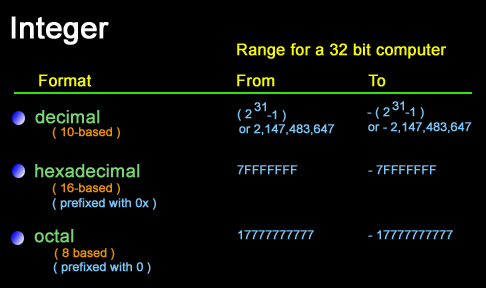# Education is not limited to just classrooms. It can be gained anytime, anywhere... - Ravi Ranjan (M.Tech-NIT)

## PHP Integers

An integer must have at least one digit (0-9). No comma or blanks are allowed within an integer.

It must not have a decimal point.

It could be either positive or negative if no sign precedes it is assumed to be positive.

Integers can be specified in three formats, decimal (10-based), hexadecimal (16-based) (prefixed with 0x) or octal (8-based) (prefixed with 0) notation, optionally preceded by a - or + sign.Example :

1. \$x = 4356; //decimal number
2. echo \$x.' is : ';
3. var_dump(\$x);
4. \$x = -39; //a negative number
5. echo '
'.\$x.' is : ';
6. var_dump(\$x);
7. \$x = 057; //octal number (equivalent to 47 decimal)
8. echo '
'.\$x.' is : ';
9. var_dump(\$x);
10. \$x = 0x4B; //hexadecimal number (equivalent to 75
11. //decimal)
12. echo '
'.\$x.' is : ';
13. var_dump(\$x);
14. ?>

View the example in browser

Output :

4356 is : int(4356)
-39 is : int(-39)
47 is : int(47)
75 is : int(75)

Note : var_dump() function is used to get structured information (type and value) about variables.

PHP integers : Range

The size and range of an integer are platform depended. For a 32 bit computer maximum number the largest integer is 231-1 or 2,147,483,647 and the smallest integer is -(231-1) or -2,147,483,647. if we specify a number beyond the range of the integer type, it will be interpreted as a float. Integer size can be determined from PHP_INT_SIZE, maximum value from PHP_INT_MAX. If you specify a number beyond the bounds of the integer type, it will be interpreted as a float instead.

Floating point numbers

Description

Floating point numbers could be written in two forms, fractional and exponential form.

Fractional form :

The number must have at least one digit (0-9). No comma or blanks are allowed within an integer. It must have a decimal point. It could be either positive or negative, default sign is positive.

Exponential form :

The exponent part is an "e" or "E" followed by an integer, which can be signed (preceded by "+" or "-").

Version

(PHP 4 and above)

Example :

1. \$a = 5.205;
2. \$b = 1.3e3;
3. \$c = 6E-10;
4. ?>